# Texas Go Math Grade 6 Lesson 10.2 Answer Key Prime Factorization

Refer to our Texas Go Math Grade 6 Answer Key Pdf to score good marks in the exams. Test yourself by practicing the problems from Texas Go Math Grade 6 Lesson 10.2 Answer Key Prime Factorization.

## Texas Go Math Grade 6 Lesson 10.2 Answer Key Prime Factorization

List all the factors of each number.

Question 1.
21 ______________
List the factors of 21
21 = 1 × 21
21 = 3 × 7
The factors of 21 are 1, 3, 7, 21.

Question 2.
37 ______________
List the factors of 37
37 = 1 × 37
The factors of 37 are 1, 37,

Question 3.
42 ______________
List the factors of 42

• 42 = 1 × 42
• 42 = 2 × 21
• 42 = 3 × 14
• 42 = 6 × 7

The factors of 42 are 1, 2, 3, 6, 7, 14, 21, 42.

Question 4.
30 ______________
List the factors of 30

• 30 = 1 × 30
• 30 = 2 × 15
• 30 = 3 × 10
• 30 = 5 × 6

The factors of 30 are 1, 2, 3, 5, 6, 10, 15, 30

Reflect

Question 5.
What If? What will the factor tree for 240 look like if you start the tree with a different factor pair? Check your prediction by creating another factor tree for 240 that starts with a different factor pair.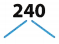Prime factorization of 240 using factor tree.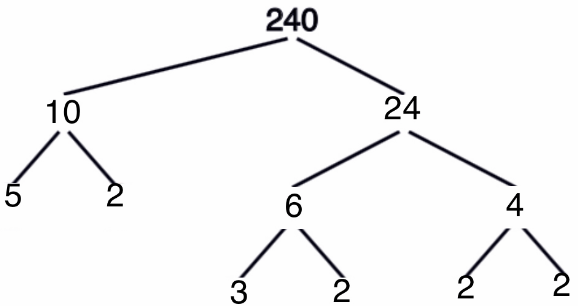Prime factorization of 240 using factor tree.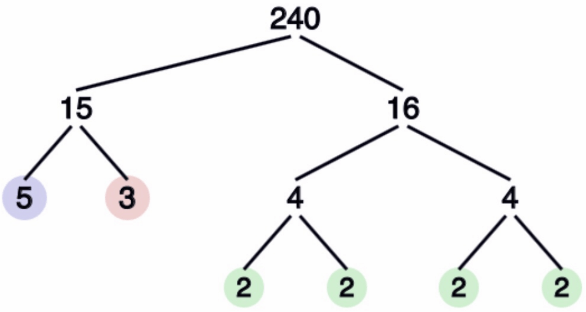The prime factors of 240 are 5 ∙ 3 ∙ 2 ∙ 2 ∙ 2 ∙ 2 or 5 ∙ 3 ∙ 24

Reflect

Question 6.
Complete a factor tree and a ladder diagram to find the prime factorization of 54.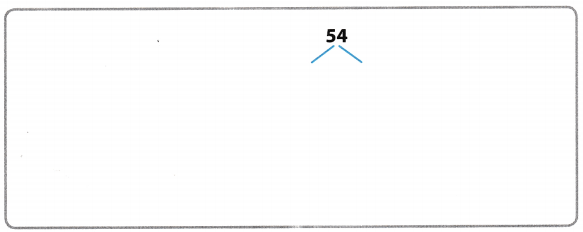Prime factorization of 54 using factor tree.Prime factorization of 54 using a ladder diagram.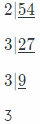The prime factors of 54 are 2 ∙ 3 ∙ 3 ∙ 3 or 2 ∙ 33

Question 7.
Communicate Mathematical Ideas If one person uses a ladder diagram and another uses a factor tree to write a prime factorization, will they get the same result? Explain.
If one person uses a ladder diagram and another uses a factor tree to write a prime factorization, they will get the same result because the prime factors of a number are identical an independent of the method used to evaluate them.

Use a diagram to list the factor pairs of each number.

Question 1.
18
Given number: 18

Write the given number as a product of its factors, therefore:

• 18 = 2 × 9
• 18 = 3 × 6

The factors of 18 are 1, 2, 3, 6, 9 and 18.

Question 2.
52
Given number: 52

Write the given number as a product of its factors, therefore:
52 = 2 × 26
52 = 4 × 13
The factors are of 52 are 1, 2, 4, 13, 26 and 52.

Question 3.
Karl needs to build a stage that has an area of 72 square feet. The length of the stage should be longer than the width. What are the possible whole number measurements for the length and width of the stage?
Complete the table with possible measurements of the stage.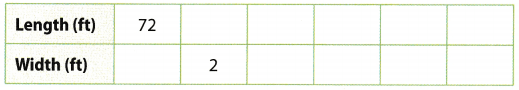Possible measurements of the stage.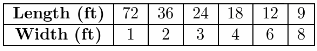List the possible measurements of the stage based on the factors of 72.

Use a factor tree to find the prime factorization of each number.

Question 4.
402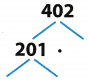Prime factorization of 402 using a factor tree.
First line of factor tree 6 × 67 = 402
Factor out 6 and you will have 3 × 2.
The complete prime factors of 402 is 3 ∙ 2 ∙ 67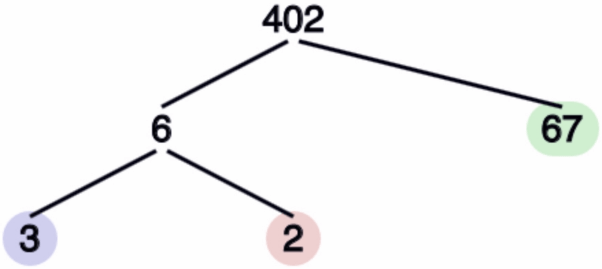Another way to show the prime factorization of 402 using a factor tree.
First line of factor tree 2 × 201 = 402
Factor out 201 and you will have 3 × 67.
The complete prime factors of 402 is 2 ∙ 3 ∙ 67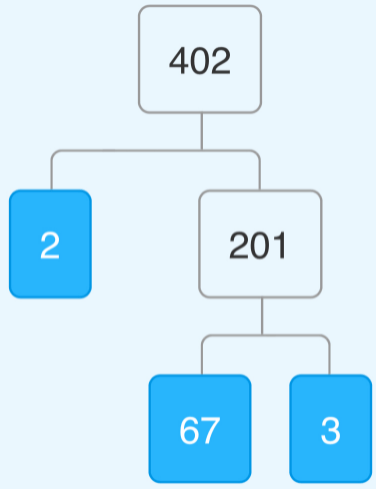Prime factors of 402 are 3 ∙ 2 67.

Question 5.
36
Prime factorization of 36 using a factor tree.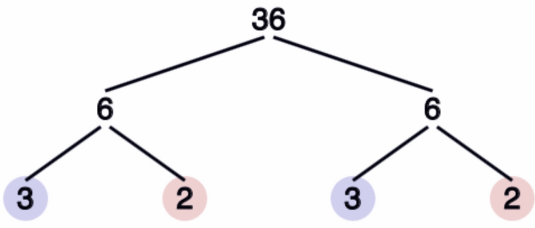Prime factors of 36 are 3 ∙ 2 ∙ 3 ∙ 2 or 32 ∙ 22

Use a ladder diagram to find the prime factorization of each number.

Question 6.
32
Determine the prime factors of 32 using continuous division.Prime factors of 32 are 2 ∙ 2 ∙ 2 ∙ 2 ∙ 2 or 25

Question 7.
27
Determine the prime factors of 27 using continuous division.The prime factors of 27 are 3 ∙ 3 ∙ 3 or 33

Essential Question Check-In

Question 8.
Tell how you know when you have found the prime factorization of a number.
The prime factorization of a number is verified by studying its factors. They must all be prime numbers.

Question 9.
Multiple Representations Use the grid to draw three different rectangles so that each has an area of 12 square units and they all have different widths. What are the dimensions of the rectangles?The dimensions of the rectangle are:
a. 1 by 12
b. 2 by 6
c. 3 by 4

Diagram of the rectangle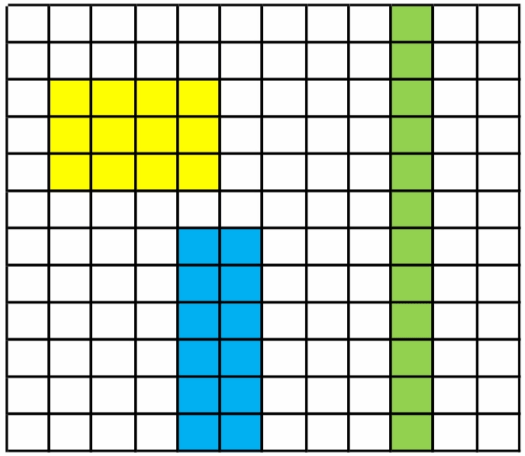Dimensions are: 1 by 12, 2 by 6, 3 by 4.

Question 10.
Brandon has 32 stamps. He wants to display the stamps in rows, with the same number of stamps in each row. How many different ways can he display the stamps? Explain.
Given Number = 32

Write the given number as a product of its factors, therefore:
32 = 2 × 16
32 = 4 × 8
This implies that he can arrange the stamps in 4 different ways. He can make 2 columns each containing 16 stamps or 2 rows each containing 16 stamps Or he can make 4 columns each containing 8 stamps or 4 rows each containing 8 stamps.

He can arrange the stamps in 4 different ways. He can make 2 columns each containing 16 stamps or 2 rows each containing 16 stamps. Or he can make 4 columns each containing 8 stamps or 4 rows each containing 8 stamps.

Question 11.
Communicate Mathematical Ideas How is finding the factors of a number different from finding the prime factorization of a number?
Finding the factors of a number indicates all the factors that may be used for the number which can be a combination of prime numbers and composite numbers. Finding the prime factorization of a number breaks a composite number into product of its prime factors.

Example:
12 – factors are 1 and 12, 2 and 6, 3 and 4
12 – prime factors are 2 ∙ 2 ∙ 3 or 22 ∙ 3

Factors are the numbers to be multiplied.
Prime factorization results to product of prime factors of the number.

Find the prime factorization of each number.

Question 12.
891 ______________
The prime factorization of a number is the number written as the product of its prime factors. For example, the prime factors of 891 are 3, 3, 3, 3 and 11.

The prime factorization of 891 is:
891 = 3 × 3 × 3 × 3 × 11 or 34 × 11
= 34 × 11

Question 13.
5.4 ______________
The prime factorization of a number is the number written as the product of its prime factors. For example, the prime factors of 504 are 2, 2, 2, 3, 3 and 7.

The prime factorization of 504 is:
504 = 2 × 2 × 2 × 3 × 3 × 7 or 23 × 32 × 7
= 23 × 32 × 7

Question 14.
23 ______________
The prime factorization of a number is the number written as the product of its prime factors. For example, the prime factors of 23 are 1 and 23.

The prime factorization of 23 is:
23 = 1 × 23

Question 15.
230 ______________
The prime factorization of a number is the number written as the product of its prime factors. For example, the prime factors of 230 are 2, 5 and 23.

The prime factorization of 230 is:
230 = 2 × 5 × 23

Question 16.
The number 2 is chosen to begin a ladder diagram to find the prime factorization of 66. What other numbers could have been used to start the ladder diagram for 66? How does starting with a different number change the diagram?
Prime factorization of 66 using ladder diagram.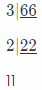It will only change the order or arrangement of the prime factors but the prime factors are still the same.
The number 3 can be used to start the diagram however the prime factors will still be the same.

Question 17.
Critical Thinking List five numbers that have 3, 5, and 7 as prime factors.

• 105 = 3 ∙ 5 ∙ 7
• 315 = 3 ∙ 3 ∙ 5 ∙ 7
• 525 = 3 ∙ 5 ∙ 5 ∙ 7
• 735 = 3 ∙ 5 ∙ 7 ∙ 7
• 1,575 = 3 ∙ 3 ∙ 5 ∙ 5 ∙ 7

The numbers are 105, 315, 525, 735, and 1,575.

Question 18.
In a game, you draw a card with three consecutive numbers on it. You can choose one of the numbers and find the sum of its prime factors. Then you can move that many spaces. You draw a card with the numbers 25, 26, 27. Which number should you choose if you want to move as many spaces as possible? Explain.
Given number:
25, 26, 27

Write the given numbers as a product of their prime factors, therefore:

• 25 = 5 × 5
• 26 = 2 × 13
• 27 = 3 × 3 × 3

Evaluate the sum of the prime factors, therefore:

• 5 + 5 = 10
• 2 + 13 = 15
• 3 × 3 × 3 = 9

It can be seen that 15 > 10 > 9 therefore the choice of the number 26 will result in the maximum number of moves.

Question 19.
Explain the Error When asked to write the prime factorization of the number 27, a student wrote 9 3. Explain the error and write the correct answer.
Given number = 27
Write the given number as a product of its prime factors, therefore:
27 = 3 × 3 × 3
Prime factors of the given number is 3 . The student’s solution is incorrect because the factor 9 ¡s not a prime factor.
The student’s solution is incorrect because the factor 9 is not a prime factor.

H.O.T. Focus On Higher Order Thinking

Question 20.
Communicate Mathematical Ideas Explain why it is possible to draw more than two different rectangles with an area of 36 square units, but it is not possible to draw more than two different rectangles with an area of 15 square units. The sides of the rectangles are whole numbers.
Given number = 36
Write the given number as a product of its factors, therefore:

• 36 = 2 × 18 = Rectang1e 1
• 36 = 3 × 12 = Rectangle 2
• 36 = 4 × 9 = Rectangle 3
• 36 = 36 × 1 = Rectangle 4
• 36 = 6 × 6 = Square

It can be seen that 4 rectangles with different dimensions can be made each having an area of 36 square units

Given number = 15
Write the given number as a product of its factors, therefore:

• 15 = 3 × 5 = Rectangle 1
• 15 = 15 × 1 = Rectangle 2

It can be seen that only 2 rectangles can be made here because the given area is a product of prime factors or the number itself.

Question 21.
Critique Reasoning Alice wants to find all the prime factors of the number you get when you multiply 17 ∙ 11 ∙ 13 ∙ 7. She thinks she has to use a calculator to perform all the multiplications and then find the prime factorization of the resulting number. Do you agree? Why or why not?
It can be seen that the number itself has factors that are prime numbers, so no calculator is required and the prime factors: 17, 11, 13 and 7 can directly be deduced.

The prime factors are 7, 11, 13 and 17.

Question 22.
Look for a Pattern Ryan wrote the prime factorizations shown below, If he continues this pattern, what prime factorization will he show for the number one million? What prime factorization will he show for one billion?
10 = 5 ∙ 2
100 = 52 ∙ 22
1,000 = 53 ∙ 23